## A pizza has a circumference of 50cm. Work out the diameter of the pizza

Question

A pizza has a circumference of 50cm. Work out the diameter of the pizza

in progress 0
6 months 2021-08-05T17:45:38+00:00 2 Answers 5 views 0

circumstance is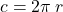so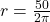r = 7.9 r= 8cm

and distance is 16cm

d =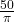50 = 2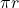50 =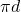d =